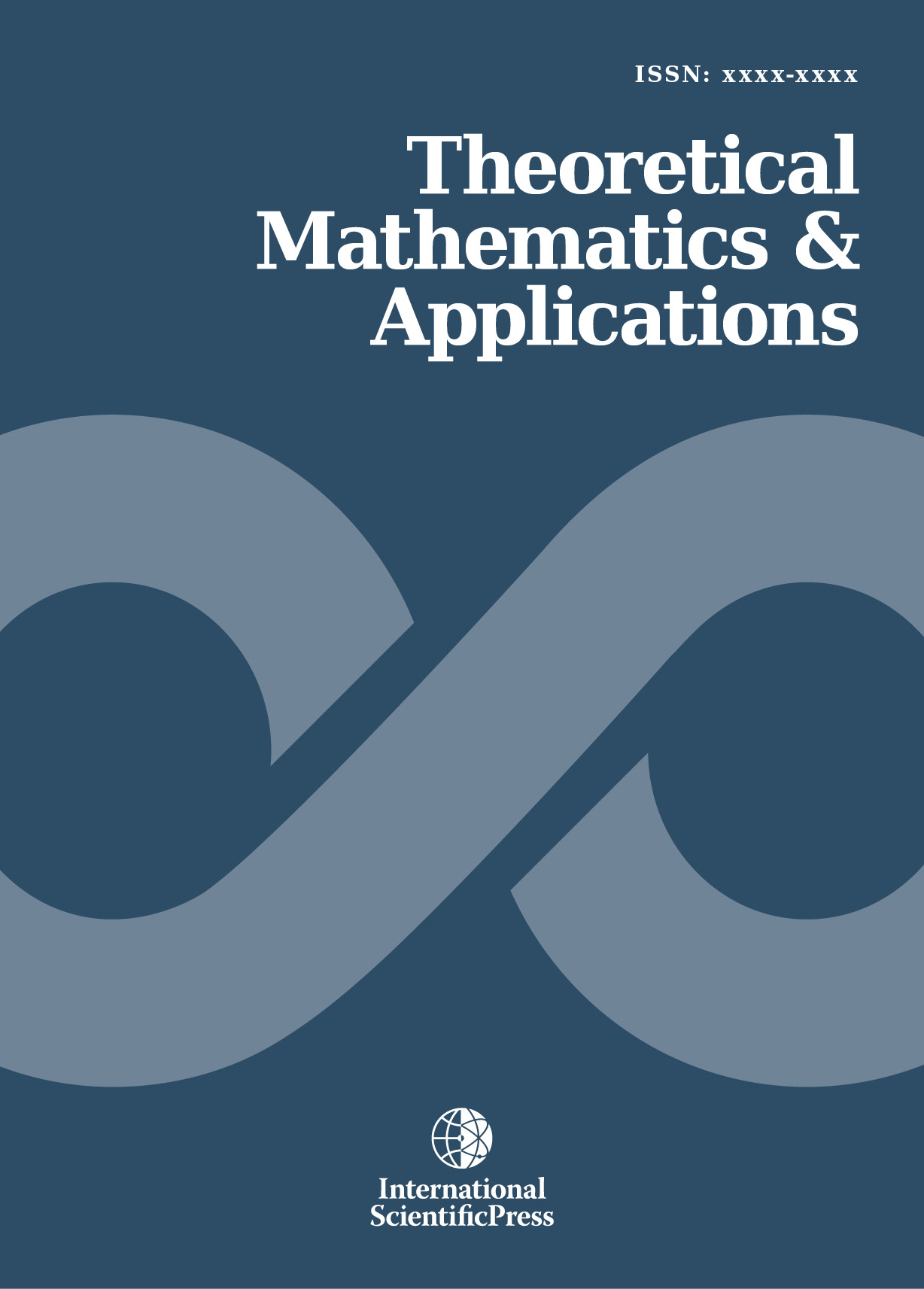# Theoretical Mathematics & Applications

#### Optimal Steady Motions for Oriented Vehicles

•[ Download ]
• Times downloaded: 1813
• Abstract

Motivated by the motion planning problem for oriented vehicles travelling in a 3-Dimensional space; Euclidean space E3  , the sphere S3 and Hyperboloid H3. For such problems the orientation of the vehicle is naturally represented by an orthonormal frame over a point in the underlying manifold. The orthonormal frame bundles of the space forms R3 , S3 and H3 correspond with their isometry groups and are the Euclidean group of motion SE(3), the rotation group SO(4) and the Lorentzian group SO(1,3) respectively. Orthonormal frame bundles of space forms coincide with their isometry groups and therefore the focus shifts to left-invariant control systems defined on Lie groups. In this paper a method for integrating these systems is given where the controls are time-independent. For constant twist motions or helical motions, the corresponding curves g(t) ε SE(3) are given in closed form by using the well known Rodrigues’ formula. However, this formula is only applicable to the Euclidean case. This paper gives a method for computing the non-Euclidean screw/helical motions in closed form. This involves decoupling the system into two lower dimensional systems using the double cover properties of Lie groups, then the lower dimensional systems are solved explicitly in closed form.ISSN: 1792-9687 (Print)
1792-9709 (Online)